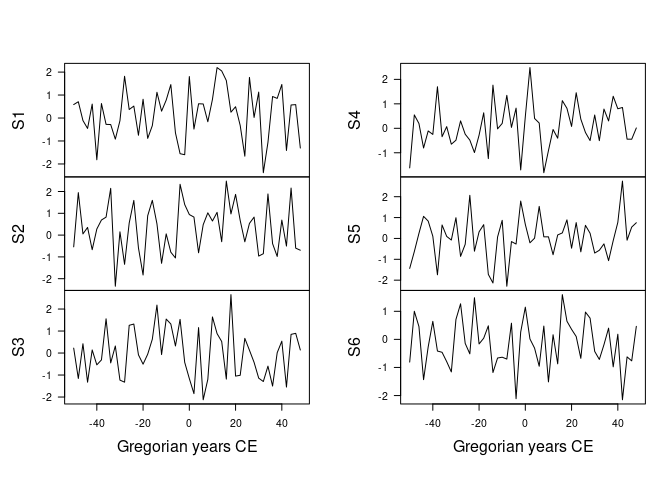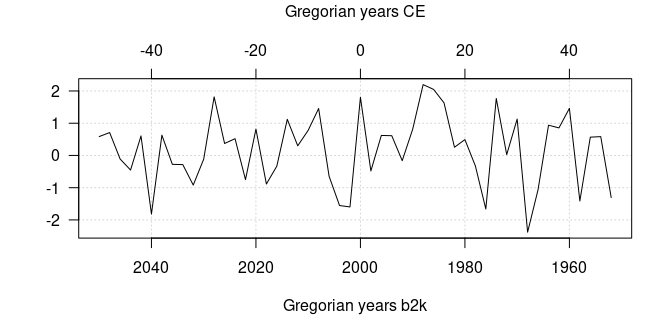# Introduction to aion

#### 2023-10-26

library(aion)

Base R ships with a lot of functionality useful for time series, in particular in the stats package. However, these features are not adapted to most archaeological time series. These are indeed defined for a given calendar era, they can involve dates very far in the past and the sampling of the observation time is (in most cases) not constant. aion provides a system of classes and methods to represent and work with such time-series.

# 1 Calendars

aion currently supports both Julian and Gregorian calendars (with the most common eras for the latter, e.g. Before Present, Common Era…). A calendar can be defined using the calendar() function:

## Create a calendar object
## (Gregorian Common Era)
calendar("CE")
#> Common Era (CE): Gregorian years counted forwards from 0.

Or by using the shortcuts:

## Common Era (Gregorian)
CE()
#> Common Era (CE): Gregorian years counted forwards from 0.

## Before Present (Gregorian)
BP()
#> Before Present (BP): Gregorian years counted backwards from 1950.

When creating date vectors or time series, you must specify the calendar corresponding to your data (see below). This allows to select the correct method for converting dates to rata die.

Outputs generated by aion are expressed in rata die by default (this can be modified on a per-function basis). The only two exceptions are the plot() and format() functions, which default to the calendar specified in the package options (see below). You can change the default calendar to be used throughout the package by modifying the aion.calendar option, or on a per-function basis.

## Get default calendar
getOption("aion.calendar")
#> Common Era (CE): Gregorian years counted forwards from 0.

## Change default calendar to BP
options(aion.calendar = BP())
getOption("aion.calendar")
#> Before Present (BP): Gregorian years counted backwards from 1950.

## Set it back to Gregorian Common Era
options(aion.calendar = CE())
getOption("aion.calendar")
#> Common Era (CE): Gregorian years counted forwards from 0.

# 2 Vectors of dates

In base R, dates are represented by default as the number of days since 1970-01-01 (Gregorian), with negative values for earlier dates (see help(Date)). aion uses a different approach: it allows to create date vectors represented as rata die (Reingold and Dershowitz 2018), i.e. as number of days since 01-01-01 (Gregorian)1.

This makes it possible to get rid of a specific calendar and to make calculations easier. It is then possible to convert a vector of rata die into dates or (decimal) years of any calendar.

The fixed() function allows to create a vector of rata die from dates belonging to a specific calendar:

## Convert 2000-02-29 (Gregorian) to rata die
fixed(2000, 02, 29, calendar = calendar("CE"))
#> Rata die: number of days since 01-01-01 (Gregorian).
#>  730179

## If days and months are missing, decimal years are expected
fixed(2000.161, calendar = calendar("CE"))
#> Rata die: number of days since 01-01-01 (Gregorian).
#>  730179

A rata die vector can be converted into dates (or years) of a particular calendar:

## Create a vector of 10 years BP (Gregorian)
## (every 20 years starting from 2000 BP)
(years <- seq(from = 20000, by = -20, length.out = 10))
#>   20000 19980 19960 19940 19920 19900 19880 19860 19840 19820

## Convert years to rata die
(rd <- fixed(years, calendar = calendar("BP")))
#> Rata die: number of days since 01-01-01 (Gregorian).
#>   -6592992 -6585687 -6578382 -6571077 -6563772 -6556467 -6549162 -6541857
#>   -6534553 -6527248

## Convert back to Gregorian years
as_year(rd, calendar = calendar("CE"))  # Common Era
#>   -18050 -18030 -18010 -17990 -17970 -17950 -17930 -17910 -17890 -17870
as_year(rd, calendar = calendar("BP"))  # Before Present
#>   20000 19980 19960 19940 19920 19900 19880 19860 19840 19820
as_year(rd, calendar = calendar("b2k")) # Before 2000
#>   20050 20030 20010 19990 19970 19950 19930 19910 19890 19870

Rata die can be represented as (nicely formated) character vectors:

format(rd) # Default calendar (Gregorian Common Era)
#>   "-18050 CE" "-18030 CE" "-18010 CE" "-17990 CE" "-17970 CE" "-17950 CE"
#>   "-17930 CE" "-17910 CE" "-17890 CE" "-17870 CE"
format(rd, prefix = "ka", calendar = calendar("BP"))
#>   "20 ka BP"    "19.98 ka BP" "19.96 ka BP" "19.94 ka BP" "19.92 ka BP"
#>   "19.9 ka BP"  "19.88 ka BP" "19.86 ka BP" "19.84 ka BP" "19.82 ka BP"

The rata die vector provides the internal time representation of the aion time-series (if you want to work with numeric vectors that represent year-based time scales, you may be interested in the era package).

# 3 Time series

A time series is a sequence of observation time and value pairs with strictly increasing observation times.

A time series object is an $$n \times m \times p$$ array, with $$n$$ being the number of observations, $$m$$ being the number of series and with the $$p$$ columns of the third dimension containing extra variables for each series. It can be created from a numeric vector, matrix or array.

## Set seed for reproductibility
set.seed(12345)

## 6 x 50 observations...
obs <- matrix(rnorm(300), nrow = 50, ncol = 6)

## ...sampled every two years starting from 2000 BP
spl <- seq(from = 2000, by = -2, length.out = 50)

## Create time series
(X <- series(object = obs, time = spl, calendar = BP()))
#> 50 x 6 x 1 time series observed between -18627 and 17167 r.d.

Time series terminal and sampling times can be retrieved and expressed according to different calendars (remember that outputs are expressed in rata die by default):

## Time series duration
span(X) # Default: rata die
#>  35794
span(X, calendar = CE())
#>  98.99726

## Time of first observation
start(X) # Default: rata die
#>  -18627
start(X, calendar = CE())
#>  -50

## Time of last observation
end(X) # Default: rata die
#>  17167
end(X, calendar = CE())
#>  48

## Sampling times
time(X, calendar = BP())
#>   2000 1998 1996 1994 1992 1990 1988 1986 1984 1982 1980 1978 1976 1974 1972
#>  1970 1968 1966 1964 1962 1960 1958 1956 1954 1952 1950 1948 1946 1944 1942
#>  1940 1938 1936 1934 1932 1930 1928 1926 1924 1922 1920 1918 1916 1914 1912
#>  1910 1908 1906 1904 1902

Plot one or more time series:

## Multiple plot
plot(X) # Default calendar## Extract the first series
Y <- X[, 1, ]

## Plot a single series
plot(
Y,
calendar = b2k(), # b2k time scale
panel.first = graphics::grid() # Add a grid
)
year_axis(side = 3, calendar = CE()) # Add a secondary time axis
mtext(format(CE()), side = 3, line = 3) # Add secondary axis titleNote that aion uses the astronomical notation for Gregorian years (there is a year 0).

1. It is intended that the rata die should be an integer value, but this is not enforced in the internal representation.↩︎